# نتائج البحث (7)

View
Selected filters:
• Inside Mathematics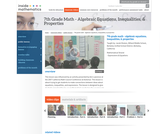Conditions of Use:
Rating

This lesson is about trying to get students to make connections between ideas about equations, inequalities, and expressions. The lesson is designed to give students opportunities to use mathematical vocabulary for a purpose to describe, discuss, and work with these symbol strings.The idea is for students to start gathering global information by looking at the whole number string rather than thinking only about individual procedures or steps. Hopefully students will begin to see the symbol strings as mathematical objects with their own unique set of attributes. (7th Grade Math)

Material Type:
Activity/Lab
Lecture
Lesson Plan
Teaching/Learning Strategy
Provider:
Noyce Foundation
Provider Set:
Inside Mathematics
Author:
Disston, Jacob
11/30/2011Conditions of Use:
Rating

This lesson is based on the results of a performance task in which we realized that students' understanding of area and perimeter was mostly procedural. Therefore the purpose of this re-engagement lesson was to address student misconceptions and deepen student understanding of area and perimeter. The standards addressed in this lesson involve finding perimeter and area of various shapes, finding the perimeter when given a fixed area, and using a formula in a practical context. Challenges for our students included decoding the language in the problem and proving their thinking. (7th Grade Math)

Material Type:
Activity/Lab
Lecture
Lesson Plan
Teaching/Learning Strategy
Provider:
Noyce Foundation
Provider Set:
Inside Mathematics
Author:
Villarin, Antoinette
11/30/2011Conditions of Use:
قراءة النص المطبوع بخط رفيع
Rating

The foundation of this lesson is constructing, communicating, and evaluating student-generated tables while making comparisons between three different financial plans. Students are given three different DVD rental plans and asked to analyze each one to see if they could determine when the 3 different DVD plans cost the same amount of money, if ever. (7th/8th Grade Math)

نوع المادة:
Activity/Lab
Lecture
Lesson Plan
Teaching/Learning Strategy
Provider:
Noyce Foundation
Provider Set:
في قلب الرياضيات
المؤلف:
Dimas, Cecilio
11/30/2011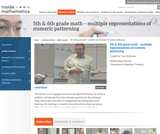Conditions of Use:
قراءة النص المطبوع بخط رفيع
Rating

This lesson is a re-engagement lesson designed for learners to revisit a problem-solving task they have already experienced. Students will activate prior knowledge of graphical representations through the 'what's my rule' number talk; compare and contrast two different learners' interpretations of the growing pattern; use multiple representations to demonstrate how one of these learners would represent the numeric pattern; make connections between the different representations to more critically compare the two interpretations. (5th/6th Grade Math)

نوع المادة:
Activity/Lab
Lecture
Lesson Plan
Teaching/Learning Strategy
Provider:
Noyce Foundation
Provider Set:
في قلب الرياضيات
المؤلف:
Dickinson, Fran
11/30/2011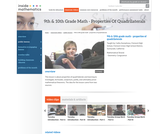Conditions of Use:
قراءة النص المطبوع بخط رفيع
Rating

This lesson is about properties of quadrilaterals and learning to investigate, formulate, conjecture, justify, and ultimately prove mathematical theorems. Students will: Analyze characteristics and properties of two- and three-dimensional geometric shapes; develop mathematical arguments about geometric relationships; and apply appropriate techniques, tools, and formulas to determine measurements.Explore relationships among classes of two- and three-dimensional geometric objects, make and test conjectures about them, and solve problems involving them. Employ forms of mathematical reasoning and proof appropriate to the solution of the problem at hand, including deductive and inductive reasoning, making and testing conjectures, and using counter examples and indirect proof. Identify, formulate and confirm conjectures. Establish the validity of geometric conjectures using deduction, prove theorems, and critique arguments made by others. (9th/10th Grade Math)

نوع المادة:
Activity/Lab
Lecture
Lesson Plan
Teaching/Learning Strategy
Provider:
Noyce Foundation
Provider Set:
في قلب الرياضيات
المؤلف:
Humphreys, Cathy
11/30/2011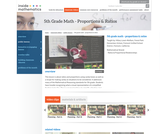Conditions of Use:
قراءة النص المطبوع بخط رفيع
Rating

This lesson is about ratios and proportions using candy boxes as well as a recipe for making candy as situations to be considered. It addresses many Mathematical Reasoning standards and asks students to: Use models to understand fractions and to solve ratio problems; think about a ratio as part/part model and to think about the pattern growing in equal groups or a unit composed of the sum of the parts; find a scale factor and apply it to a ratio. (5th Grade Math)

نوع المادة:
Activity/Lab
Lecture
Lesson Plan
Teaching/Learning Strategy
Provider:
Noyce Foundation
Provider Set:
في قلب الرياضيات
المؤلف:
Lewis-Wolfsen, Hillary
11/30/2011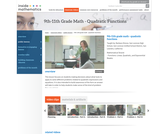Conditions of Use:
قراءة النص المطبوع بخط رفيع
Rating

This lesson focuses on students making decisions about what tools to apply to solve different problems related to quadratic expressions and equations. It is also intended to build awareness of the form an answer will take in order to help students make sense of the kind of problem they are solving. (9th/10th/11th Grade Math)

نوع المادة:
Activity/Lab
Lecture
Lesson Plan
Teaching/Learning Strategy
Provider:
Noyce Foundation
Provider Set:
في قلب الرياضيات
المؤلف:
Shreve, Barbara